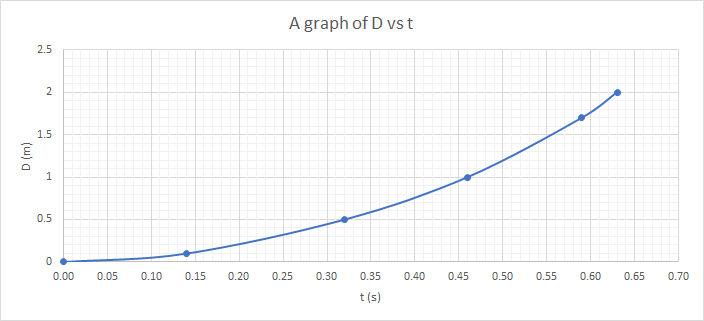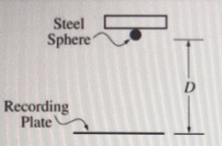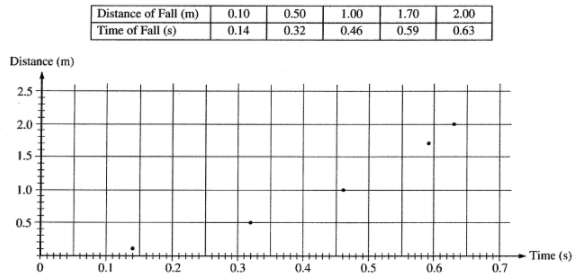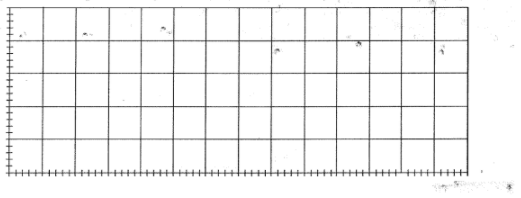# Problem: A student wishing to determine experimentally the acceleration g due to gravity has an apparatus that holds a small steel sphere above a recording plate, as shown above. When the sphere is released, a timer automatically begins recording the time of fall. The timer automatically stops when the sphere strikes the recording plate. The student measures the time of fall for different values of the distance D shown above and records the data in the table below. These data points are also plotted on the graph.(a) On the grid above, sketch the smooth curve that best represents the student’s data.The student can use these data for distance D and time t to produce a second graph from which the acceleration g due to gravity can be determined.(b) If only the variables D and t are used, what quantities should the student graph in order to produce a linear relationship between the two quantities?(c) On the grid below, plot the data points for the quantities you have identified in part (b), and sketch the best straight-line fir to the points. Label your axes and show the scale that you have chosen for the graph.(d) Using the slope of your graph in part (c), calculate the acceleration g due to gravity in this experiment.(e) State one way in which the student could improve the accuracy of the results if the experiment were to be performed again. Explain why this would improve the accuracy.

###### FREE Expert Solution

(a) To sketch the curve, we'll make points on the graph using the given information and join them using a smooth curve.The curve is as shown above. The shape tells us that the motion has a constant acceleration.

98% (251 ratings)###### Problem DetailsA student wishing to determine experimentally the acceleration g due to gravity has an apparatus that holds a small steel sphere above a recording plate, as shown above. When the sphere is released, a timer automatically begins recording the time of fall. The timer automatically stops when the sphere strikes the recording plate. The student measures the time of fall for different values of the distance D shown above and records the data in the table below. These data points are also plotted on the graph.(a) On the grid above, sketch the smooth curve that best represents the student’s data.

The student can use these data for distance D and time t to produce a second graph from which the acceleration g due to gravity can be determined.

(b) If only the variables D and t are used, what quantities should the student graph in order to produce a linear relationship between the two quantities?

(c) On the grid below, plot the data points for the quantities you have identified in part (b), and sketch the best straight-line fir to the points. Label your axes and show the scale that you have chosen for the graph.(d) Using the slope of your graph in part (c), calculate the acceleration g due to gravity in this experiment.

(e) State one way in which the student could improve the accuracy of the results if the experiment were to be performed again. Explain why this would improve the accuracy.